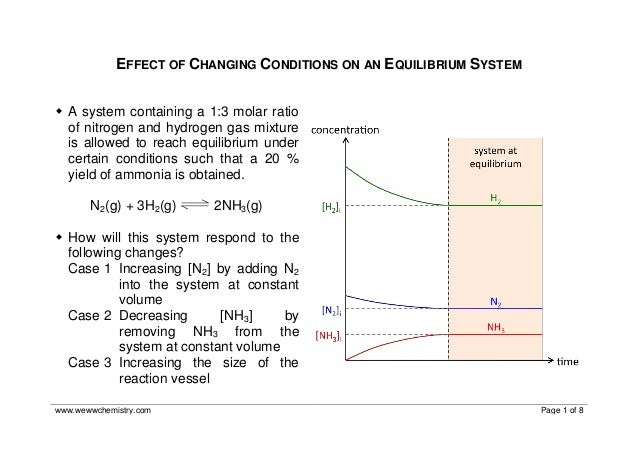Successfully reported this slideshow.Upcoming SlideShare
×

# Effect of Changing Conditions on an Equilibrium System

2,353 views

Published on

[ Related blog post: http://www.wewwchemistry.com/2013/04/chemical-equilibria ] This worked example illustrates graphically the effect of changing certain reaction conditions on an equilibrium. It is prepared to complement my Chemical Equilibria Summary slides and describes in greater detail, how the concentration-time graphs on Slides 29, 32 and 36 were derived.

• Full Name
Comment goes here.

Are you sure you want to Yes No• Be the first to comment

• Be the first to like this

### Effect of Changing Conditions on an Equilibrium System

1. 1. www.wewwchemistry.com Page 1 of 8 EFFECT OF CHANGING CONDITIONS ON AN EQUILIBRIUM SYSTEM  A system containing a 1:3 molar ratio of nitrogen and hydrogen gas mixture is allowed to reach equilibrium under certain conditions such that a 20 % yield of ammonia is obtained. N2(g) + 3H2(g) 2NH3(g)  How will this system respond to the following changes? Case 1 Increasing [N2] by adding N2 into the system at constant volume Case 2 Decreasing [NH3] by removing NH3 from the system at constant volume Case 3 Increasing the size of the reaction vessel
2. 2. www.wewwchemistry.com Page 2 of 8 Case 1: Increasing [N2] by adding N2 into the system at constant volume By Le Chatelier’s Principle, equilibrium position shifts right to decrease [N2]. As system re-establishes equilibrium, both [N2] and [NH3] increase while [H2] decreases relative to their initial concentrations before N2 was added. N2 + 3H2 → 2NH3 [ ] before N2 is added / mol dm–3 (system is at initial equilibrium) [N2]i [H2]i [NH3]i [ ] after N2 is added / mol dm–3 (equilibrium is disturbed) [N2]i,new (> [N2]i) [H2]i [NH3]i Change in [ ] / mol dm–3 (system responds to change) –x –3x +2x New equilibrium [ ] / mol dm–3 (system establishes a new equilibrium) [N2]i,new – x (> [N2]i) [H2]i – 3x (< [H2]i) [NH3]i + 2x (> [NH3]i)
3. 3. www.wewwchemistry.com Page 3 of 8
4. 4. www.wewwchemistry.com Page 4 of 8 Case 2: Decreasing [NH3] by removing NH3 from the system at constant volume By Le Chatelier’s Principle, equilibrium position shifts right to increase [NH3]. As system re-establishes equilibrium, the final [N2], [H2] and [NH3] are all lower than their initial concentrations before NH3 was removed. N2 + 3H2 → 2NH3 [ ] before NH3 is removed / mol dm–3 (system is at initial equilibrium) [N2]i [H2]i [NH3]i [ ] after NH3 is removed / mol dm–3 (equilibrium is disturbed) [N2]i [H2]i [NH3]i,new (< [NH3]i) Change in [ ] / mol dm–3 (system responds to change) –x –3x +2x Equilibrium [ ] / mol dm–3 (system establishes a new equilibrium) [N2]i – x (< [N2]i) [H2]i – 3x (< [H2]i) [NH3]i,new + 2x (< [NH3]i)
5. 5. www.wewwchemistry.com Page 5 of 8
6. 6. www.wewwchemistry.com Page 6 of 8 Case 3: Increasing the size of the reaction vessel This decreases gas pressure in the system, and all concentrations decrease. According to Le Chatelier’s Principle, equilibrium position shifts left to favour the side of the reaction with a larger number of gas molecules. After system re-establishes equilibrium, the final [N2], [H2] and [NH3] are all lower than their initial concentrations before the size of the reaction vessel was increased. Note that [NH3] decreases to a larger extent than [N2] and [H2].
7. 7. www.wewwchemistry.com Page 7 of 8 N2 + 3H2 → 2NH3 [ ] before N2 is added / mol dm–3 (system is at initial equilibrium) [N2]i [H2]i [NH3]i [ ] after size of reaction vessel is increased / mol dm–3 (equilibrium is disturbed) [N2]i,new (< [N2]i) [H2]i,new (< [H2]i) [NH3]i,new (< [NH3]i) Change in [ ] / mol dm–3 (system responds to change) +x +3x –2x Equilibrium [ ] / mol dm–3 (system establishes a new equilibrium) [N2]i,new + x (< [N2]i) [H2]i,new + 3x (< [H2]i) [NH3]i,new – 2x (< [NH3]i)
8. 8. www.wewwchemistry.com Page 8 of 8